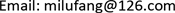﻿ 一类广义的带惯性项的Cahn-Hilliard方程的行波解 The Traveling Wave Solutions for a Class of Generalized Cahn-Hilliard Equation with Inertia Term

Vol. 08  No. 05 ( 2019 ), Article ID: 30228 , 5 pages
10.12677/AAM.2019.85101

The Traveling Wave Solutions for a Class of Generalized Cahn-Hilliard Equation with Inertia Term

Chuanye Zhao1, Yanmei Wang2, Lufang Mi1

1College of Science, Binzhou University, Binzhou Shandong

2Second Experimental Primary School of Zhanhua District, Binzhou ShandongReceived: Apr. 25th, 2019; accepted: May 9th, 2019; published: May 16th, 2019ABSTRACT

Cahn-Hilliard equation is a kind of important non-linear diffusion equation in mathematical physics. In this paper, we consider a class of generalized Cahn-Hilliard equations with inertia terms. The traveling wave solution of this kind of equation is further explored. The exact traveling wave solutions of the equation are obtained by using the hyperbolic tangent function method, and these traveling wave solutions have the properties of shock waves.

Keywords:Traveling Wave Solutions, Cahn-Hilliard Equation, The Hyperbolic Tangent Function Method

1滨州学院理学院，山东 滨州

2沾化区第二实验小学，山东 滨州Cahn-Hilliard型方程是数学物理方程中一类重要的非线性扩散方程。本文考虑一类广义的带惯性项的Cahn-Hilliard方程，对这类方程的行波解问题作了进一步的探究。利用双曲正切函数法得到了方程的精确行波解，并知道这类行波解具有激波的性质。1. 引言

Cahn-Hilliard型方程是一类重要的高阶非线性偏微分方程，它来源于自然界中广泛存在的扩散现象，最早研究这类方程的是Cahn和Hilliard，之后在很多的物理现象、生物现象中也出现了此类方程的模型。例如：热力学中两种物质之间相互扩散现象  ，生物种群的竞争与排斥现象  ，河床迁移的过程  ，固体表面上微滴的扩散  等。在过去的几十年中，特别是近二十年来，国内外众多的学者对这类方程进行了广泛的研究，但真正对Cahn-Hilliard方程系统的研究是在八十年代开始的。首先对反应扩散方程    进行了分析讨论，他们把许多物理、化学以及生物等领域的许多问题，通过分析这些转变过程，在假设摩擦比较小的情况下，具有以下几个特征：波传播的速度有限，有限振动以及传播过程中波的形状几乎不发生变化这样都可以抽象成反应扩散方程进行研究。通过研究反应扩散方程，发现其有一类特殊的形式不变解，也就是现在要研究的行波解，行波解的稳定性、唯一性可以很好的解释物质能量的传播扩散过程。行波解的模型在物理中有很多的用处，对描述物体状态的变化具有重要作用。因此，得到方程的精确解，对分析数学物理中的非线性问题提供了简单有效的方法。对Cahn-Hilliard方程的行波解的存在性、正则性问题，已有很多的方法，但对于求方程的精确行波解，利用双曲正切函数法是解决这类问题的常用方法  。因此，本文主要借助Maple软件利用双曲正切函数法来求带惯性项的一类Cahn-Hilliard方程的行波解。

2. Cahn-Hilliard方程及行波变换

$\eta {u}_{tt}+{u}_{t}+{u}_{xxxx}-{u}^{2}=0$$\eta$ 为正常数， $x\in R$ (1)

$u\left(x,t\right)$ 是关于 $x,t$ 的实值函数，为了得到该方程的行波解，对该方程进行转化。引进波变量 $\xi =c\left(x-\lambda t\right)$，其中c为波数， $\lambda$ 为波速，令

$u\left(x,t\right)=U\left(\xi \right)$ (2)

(2)是方程(1)的行波解，则有

$\left\{\begin{array}{l}{u}_{t}=-c\lambda {U}^{\prime }\left(\zeta \right),\\ {u}_{tt}={c}^{2}{\lambda }^{2}{U}^{″}\left(\zeta \right),\\ {u}_{x}=c{U}^{\prime }\left(\zeta \right),\\ {u}_{xx}={c}^{2}{U}^{″}\left(\zeta \right),\\ {u}_{xxx}={c}^{3}{U}^{‴}\left(\zeta \right),\\ {u}_{xxxx}={c}^{4}{U}^{\left(4\right)}\left(\zeta \right),\end{array}$ (3)

$\eta {c}^{2}{\lambda }^{2}{U}^{″}\left(\zeta \right)-c\lambda {U}^{\prime }\left(\zeta \right)+{c}^{4}{U}^{\left(4\right)}\left(\zeta \right)-{U}^{2}\left(\zeta \right)=0$ (4)

3. Tanh函数法求行波解

$Y=\mathrm{tanh}\left(\zeta \right)$

$U\left(\zeta \right)=S\left(Y\right)=\underset{k=0}{\overset{N}{\sum }}{a}_{k}{Y}^{k}$ (5)

${U}^{\prime }\left(\zeta \right)=\left(1-{Y}^{2}\right){S}^{\prime }\left(Y\right)$ (6)

${U}^{″}\left(\zeta \right)=\left(1-{Y}^{2}\right)\left[-2Y{S}^{\prime }\left(Y\right)+\left(1-{Y}^{2}\right){S}^{″}\left(Y\right)\right]$ (7)

${U}^{‴}\left(\zeta \right)={\left(1-{Y}^{2}\right)}^{3}{S}^{‴}\left(Y\right)-16Y{\left(1-{Y}^{2}\right)}^{2}{S}^{″}\left(Y\right)-2\left(1-{Y}^{2}\right)\left(3{Y}^{2}-1\right){S}^{\prime }\left(Y\right)$ (8)

$\begin{array}{c}{U}^{\left(4\right)}\left(\zeta \right)={\left(1-{Y}^{2}\right)}^{4}{S}^{\left(4\right)}\left(Y\right)-12Y{\left(1-{Y}^{2}\right)}^{3}{S}^{‴}\left(Y\right)+4{\left(1-{Y}^{2}\right)}^{2}\left(2{Y}^{2}+7Y-2\right){S}^{″}\left(Y\right)\\ \text{\hspace{0.17em}}\text{\hspace{0.17em}}+8Y\left(1-{Y}^{2}\right)\left(2-3{Y}^{2}\right){S}^{\prime }\left(Y\right)\end{array}$ (9)

$\begin{array}{l}\eta {c}^{2}{\lambda }^{2}\left(1-{Y}^{2}\right)\left[-2Y{S}^{\prime }\left(Y\right)+\left(1-{Y}^{2}\right){S}^{″}\left(Y\right)\right]-c\lambda \left(1-{Y}^{2}\right){S}^{\prime }\left(Y\right)\\ \text{ }+{c}^{4}{\left(1-{Y}^{2}\right)}^{4}{S}^{\left(4\right)}\left(Y\right)-12{c}^{4}Y{\left(1-{Y}^{2}\right)}^{3}{S}^{‴}\left(Y\right)+4{c}^{4}{\left(1-{Y}^{2}\right)}^{2}\left(2{Y}^{2}+7Y-2\right){S}^{″}\left(Y\right)\\ \text{ }+8{c}^{4}Y\left(1-{Y}^{2}\right)\left(2-3{Y}^{2}\right){S}^{\prime }\left(Y\right)-{S}^{2}\left(Y\right)=0\end{array}$ (10)

$order\left(U\right)=N$

$order\left({u}_{xxxx}\right)=N+4$

$order\left({u}^{2}\right)=2N$

$N+4=2N$

$S\left(Y\right)=\underset{k=0}{\overset{N}{\sum }}{a}_{k}{Y}^{k}={a}_{0}+{a}_{1}Y+{a}_{2}{Y}^{2}+{a}_{3}{Y}^{3}+{a}_{4}{Y}^{4}$

${S}^{\prime }\left(Y\right)={a}_{1}+2{a}_{2}Y+3{a}_{3}{Y}^{2}+4{a}_{4}{Y}^{3}$ (11)

${S}^{″}\left(Y\right)=2{a}_{2}+6{a}_{3}Y+12{a}_{4}{Y}^{2}$ (12)

${S}^{‴}\left(Y\right)=6{a}_{3}+24{a}_{4}Y$ (13)

${S}^{\left(4\right)}\left(Y\right)=24{a}_{4}$ (14)

$\begin{array}{l}\eta {c}^{2}{\lambda }^{2}\left(1-{Y}^{2}\right)\left[-2Y\left({a}_{1}+2{a}_{2}Y+3{a}_{3}{Y}^{2}+4{a}_{4}{Y}^{3}\right)+\left(1-{Y}^{2}\right)\left(2{a}_{2}+6{a}_{3}Y+12{a}_{4}{Y}^{2}\right)\right]\\ \text{ }-c\lambda \left(1-{Y}^{2}\right)\left({a}_{1}+2{a}_{2}Y+3{a}_{3}{Y}^{2}+4{a}_{4}{Y}^{3}\right)+24{c}^{4}{\left(1-{Y}^{2}\right)}^{4}{a}_{4}-12{c}^{4}Y{\left(1-{Y}^{2}\right)}^{3}\left(6{a}_{3}+24{a}_{4}Y\right)\\ \text{ }+4{c}^{4}{\left(1-{Y}^{2}\right)}^{2}\left(2{Y}^{2}+7Y-2\right)\left(2{a}_{2}+6{a}_{3}Y+12{a}_{4}{Y}^{2}\right)+8{c}^{4}Y\left(1-{Y}^{2}\right)\left(2-3{Y}^{2}\right)\left({a}_{1}+2{a}_{2}Y+3{a}_{3}{Y}^{2}+4{a}_{4}{Y}^{3}\right)\\ -{\left({a}_{0}+{a}_{1}Y+{a}_{2}{Y}^{2}+{a}_{3}{Y}^{3}+{a}_{4}{Y}^{4}\right)}^{2}=0\end{array}$

${Y}^{0}$ 的系数： $2\eta {c}^{2}{\lambda }^{2}{a}_{2}+c\lambda {a}_{1}+24{c}^{4}{a}_{4}-16{c}^{4}{a}_{2}+{c}^{4}{a}_{0}^{2}=0$

${Y}^{1}$ 的系数： $-2\eta {c}^{2}{\lambda }^{2}{a}_{1}+6\eta {c}^{2}{\lambda }^{2}{a}_{3}+2c\lambda {a}_{2}-120{c}^{4}{a}_{3}+56{c}^{4}{a}_{2}+16{c}^{4}{a}_{1}+{c}^{4}{a}_{1}^{2}+2{c}^{4}{a}_{0}{a}_{2}=0$

${Y}^{2}$ 的系数： $-8\eta {c}^{2}{\lambda }^{2}{a}_{2}+12\eta {c}^{2}{\lambda }^{2}{a}_{4}+3c\lambda {a}_{3}-c\lambda {a}_{1}-480{c}^{4}{a}_{4}+80{c}^{4}{a}_{2}+168{c}^{4}{a}_{3}+{c}^{4}{a}_{1}{}^{2}+2{c}^{4}{a}_{0}{a}_{2}=0$

${Y}^{3}$ 的系数： $-18\eta {c}^{2}{\lambda }^{2}{a}_{3}+2\eta {c}^{2}{\lambda }^{2}{a}_{1}+4c\lambda {a}_{4}-2c\lambda {a}_{2}+408{c}^{4}{a}_{3}+1200{c}^{4}{a}_{4}-112{c}^{4}{a}_{2}-40{c}^{4}{a}_{1}+2{c}^{4}{a}_{0}{a}_{3}+2{c}^{4}{a}_{1}{a}_{2}=0$

${Y}^{4}$ 的系数： $-32\eta {c}^{2}{\lambda }^{2}{a}_{4}+6\eta {c}^{2}{\lambda }^{2}{a}_{2}-3c\lambda {a}_{3}+1360{c}^{4}{a}_{4}-112{c}^{4}{a}_{2}-336{c}^{4}{a}_{3}+{c}^{4}{a}_{2}^{2}+2{c}^{4}{a}_{0}{a}_{4}+2{c}^{4}{a}_{1}{a}_{3}=0$

${Y}^{5}$ 的系数： $12\eta {c}^{2}{\lambda }^{2}{a}_{3}-4c\lambda {a}_{4}-432{c}^{4}{a}_{3}-672{c}^{4}{a}_{4}+56{c}^{4}{a}_{2}+24{c}^{4}{a}_{1}+2{c}^{2}{a}_{1}{a}_{4}+2{c}^{4}{a}_{2}{a}_{3}=0$

${Y}^{6}$ 的系数： $20\eta {c}^{2}{\lambda }^{2}{a}_{4}-1312{c}^{4}{a}_{4}+64{c}^{4}{a}_{2}+168{c}^{4}{a}_{3}+{c}^{4}{a}_{3}{}^{2}+2{c}^{4}{a}_{2}{a}_{4}=0$

${Y}^{7}$ 的系数： $192{c}^{4}{a}_{3}+336{c}^{4}{a}_{4}+2{c}^{4}{a}_{3}{a}_{4}=0$

${Y}^{8}$ 的系数： $504{c}^{4}{a}_{4}+{c}^{4}{a}_{4}^{2}=0$

$\lambda =\sqrt{\frac{3\eta -2}{{\eta }^{2}}},c=\sqrt{\frac{3\eta -2}{400}}$

${a}_{0}=110.1137,{a}_{1}=0.0000,{a}_{2}=0.0000,{a}_{3}=-207.5346,{a}_{4}=-504.0000$

$\begin{array}{c}u\left(x,t\right)=110.1137-207.5346{\left[\mathrm{tanh}\left(\sqrt{\frac{3\eta -2}{400}}\left(x-\sqrt{\frac{3\eta -2}{{\eta }^{2}}}t\right)\right)\right]}^{3}\\ \text{\hspace{0.17em}}\text{\hspace{0.17em}}-504.0000{\left[\mathrm{tanh}\left(\sqrt{\frac{3\eta -2}{400}}\left(x-\sqrt{\frac{3\eta -2}{{\eta }^{2}}}t\right)\right)\right]}^{4}\end{array}$ (15)

$\begin{array}{c}u\left(x,t\right)=110.1137-207.5346{\left[\mathrm{coth}\left(\sqrt{\frac{3\eta -2}{400}}\left(x-\sqrt{\frac{3\eta -2}{{\eta }^{2}}}t\right)\right)\right]}^{3}\\ \text{\hspace{0.17em}}\text{\hspace{0.17em}}-504.0000{\left[\mathrm{coth}\left(\sqrt{\frac{3\eta -2}{400}}\left(x-\sqrt{\frac{3\eta -2}{{\eta }^{2}}}t\right)\right)\right]}^{4}\end{array}$ (16)

4. 小结

The Traveling Wave Solutions for a Class of Generalized Cahn-Hilliard Equation with Inertia Term[J]. 应用数学进展, 2019, 08(05): 897-901. https://doi.org/10.12677/AAM.2019.85101

1. 1. Cahn, J.W. and Hilliard, J.E. (1958) Free Energy of Non-Uniform System Interfacial Free Energy. The Journal of Chemical Physics, 28, 258-267. https://doi.org/10.1063/1.1744102

2. 2. Coheh, D.S. and Murrary, J.D. (1991) A Generalize Diffusion Model for Growth and Dispersal in a Population. Mathematical Biology, 56, 134-137.

3. 3. Hazewinkel, M., Kaashoek, J.F. and Leynse, B. (1986) Pattern Formation for a One Dimensional Evo-lution Equation Based on Thoma River Basin Model. Mathematics & Its Applications, 30, 23-46.

4. 4. Tayler, A.B. (1986) Mathematical Models in Applied Mechanics. Clarendon, Oxford.

5. 5. 叶其孝, 李正元. 反应扩散方程引论[M]. 北京: 科学出版社, 1990.

6. 6. 盛伟杰. 双稳性反应扩散方程的非平面行波解[D]: [博士学位论文]. 兰州: 兰州大学, 2012.

7. 7. 张平安. 反应扩散方程行波解的定性研究[D]: [博士学位论文]. 兰州: 兰州大学, 2010.

8. 8. 赵才地. 非线性Cahn-Hilliard方程的行波解[J]. 武汉科技大学学报(自然科学版), 2006, 29(2): 215-216.